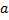# Three point massesare located at the vertices of an equilateral triangle of length ‘’. The moment of inertia of the system about an axis along the altitude of the triangle passing throughis a)b)c)d)## Question ID - 53694 :- Three point massesare located at the vertices of an equilateral triangle of length ‘’. The moment of inertia of the system about an axis along the altitude of the triangle passing throughis a)b)c)d)3537

(a)

M.I. of system about the axis which passing throughNext Question :
 Letbe three non-zero  vectors such that no two these are collinear. If the vector+2is collinear withandis collinear with(λ being some non-zero scalar). Thenequals a)b)c)d)